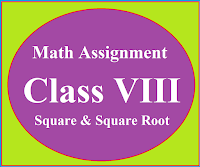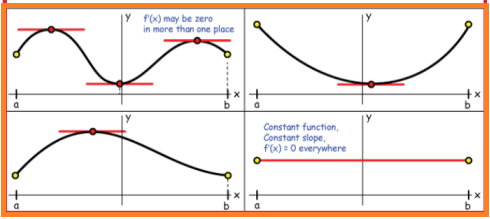### Math Assignment Class VIII | Square & Square RootMath Assignment  Class VIII | Square & Square Root Download or Print free  assignment with answer key  for   Class  8 Squares and  Square Roots.   Important and extra questions that cover all topics of square and square root and is useful and helpful for the students. Math Assignment  Class VIII | Square & Square Root LEVEL -1

# Differentiation Chapter - 5, Class 12Continuity @ Differentiability

Method of explaining continuity and differentiable, method of finding the derivative by First Principal. Differentiation of polynomial functions, logarithmic functions , exponential functions and trigonometric functions, product rule, quotient rule in differentiation

## DIFFERENTIABILITY

f(x) is said to be differentiable if left hand differentiation is equal to the right hand differentiation.
$\lim_{x\to a^{-}}\frac{f(x)-f(a)}{x-a}=\lim_{x\to a^{+}}\frac{f(x)-f(a)}{x-a}$

## DERIVATIVE BY FIRST PRINCIPAL

$f^{'}(x)=\lim_{h\to0}\frac{f(x+h)-f(x)}{h}\; (OR) \\ f^{'}(x)=\lim_{x\to a}\frac{f(x)-f(a)}{x-a}$

## PRODUCT RULE OF DIFFERENTIATION

$\left ( uv \right )^{'}=u^{'}v+uv^{'}$

## QUOTIENT RULE OF DIFFERENTIATION

$\left ( \frac{u}{v} \right )^{'}=\frac{u'v-uv'}{v^{2}}$

## DIFFERENTIATION OF TRIGONOMETRIC AND OTHER FUNCTIONS

Derivative of polynomial functions
$\frac{d}{dx}\left ( Constant \right )=0$
$\frac{d}{dx}x^{n}=nx^{n-1}$
$\frac{d}{dx}\left ( x^{5} \right )=5x^{4}$
$\frac{d}{dx}\left ( ax+b \right )=a$
$\frac{d}{dx}\left ( ax^{2}+bx \right )=2ax+b$
$\frac{d}{dx}\left ( ax^{5}+bx^{4}+cx^{3}+20 \right )=5ax^{4}+4bx^{3}+3cx^{2}$
Derivative of logarithmic functions
$\frac{d}{dx}logx=\frac{1}{x}$
$\frac{d}{dx}\left ( \frac{1}{x} \right )=-\frac{1}{x^{2}}$
$\frac{d}{dx}\left ( \frac{1}{x^{2}} \right )=-\frac{2}{x^{3}}$
$\frac{d}{dx}\left ( \frac{1}{x^{3}} \right )=-\frac{3}{x^{4}}$
Derivative of exponential functions
$\frac{d}{dx}e^{x}=e^{x}$
$\frac{d}{dx}e^{2x}=2e^{2x}$
$\frac{d}{dx}e^{5x}=5e^{5x}$
$\frac{d}{dx}e^{ax}=ae^{ax}$
$\frac{d}{dx}a^{x}=a^{x}\frac{d}{dx}x\: loga=a^{x}\: loga$
$\frac{d}{dx}2^{x}=2^{x}\frac{d}{dx}x\: log\: 2=2^{x}\: log\: 2$
Derivative of trigonometric functions
$\frac{d}{dx}sinx=cosx$
$\frac{d}{dx}cosx=-sinx$
$\frac{d}{dx}tanx=sec^{2}x$
$\frac{d}{dx}cotx=-cosec^{2}x$
$\frac{d}{dx}secx=secx\; tanx$
$\frac{d}{dx}cosecx=-cosecx\; cotx$

## DIFFERENTIATION OF INVERSE TRIGONOMETRIC FUNCTIONS

$\frac{d}{dx}sin^{-1}x=\frac{1}{\sqrt{1-x^{2}}}$
$\frac{d}{dx}cos^{-1}x=\frac{-1}{\sqrt{1-x^{2}}}$
$\frac{d}{dx}sec^{-1}x=\frac{1}{x\sqrt{x^{2}-1}}$
$\frac{d}{dx}cosec^{-1}x=\frac{-1}{x\sqrt{x^{2}-1}}$
$\frac{d}{dx}tan^{-1}x=\frac{1}{1+x^{2}}$
$\frac{d}{dx}cot^{-1}x=\frac{-1}{1+x^{2}}$

## Method of writing the derivatives

First order derivatives can be written as $\frac{dy}{dx}=y'=y_{1}$Second order derivatives can be written as $\frac{d^{2}y}{dx^{2}}=y''=y_{2}$Third order derivatives can be written as$\frac{d^{3}y}{dx^{3}}=y'''=y_{3}$

## LOGARITHMIC FUNCTIONS

Any function of type  y =   is called logarithmic function
If base b is replaced by 10 then it is called common logarithm.
If base b is replaced by 'e' then it is called natural logarithm .
Natural logarithm is denoted by lnx.
In place of lnx we simple write logx $log\: mn=log\;m+log\;n$$log\frac{m}{n}=logm-logn$$log\: m^{n}=n\: log\: m$

## ROLL'S THEOREM

If f(x) is a continuous function in [a, b] and is differentiable in (a, b) and f(a) = f(b) then there exist some c ∊ (a, b) such that f ' (c) = 0

## Algorithm for proving Roll's Theorem

a) Explain the continuity of the function f(x) in close interval [a, b]

b) Explain the differentiable of the function f(x) in open interval (a, b). If function is differentiable then find f '(x).

c) Check whether f(a) = f(b)

d) If all the above three conditions are satisfied then there exist some c ∊ (a, b) such that f ' (c) = 0.
Use this equation to find the value of c.

If  c ∊ (a, b) then Roll's Theorem verified.

NOTE:  f '(x) is called the slope of tangent and when f '(x) = 0 then slope is become parallel to the x-axis.
In the figure below  red lines shows the tangents are parallel to the x-axis at all the points where f '(x) = 0## MEAN VALUE THEOREM

If f(x) is a continuous function in [a,b] and is differentiable in (a,b) then there exist some c ∊ (a, b)  such that    $f'(c)=\frac{f(b)-f(a)}{b-a}$

## Algorithm for proving Mean Value Theorem

a) Explain the continuity of the function f(x) in close interval [a, b]

b) Explain the differentiable of the function f(x) in open interval (a, b). If function is differentiable then find f '(x).

c) When both the above conditions are satisfied the use  formula given below to find the value of c. $f'(c)=\frac{f(b)-f(a)}{b-a}$
If  c ∊ (a, b) then Mean Value Theorem is verified.